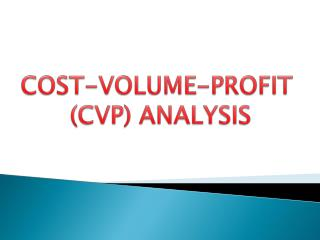Download PresentationCOST-VOLUME-PROFIT (CVP) ANALYSIS

# COST-VOLUME-PROFIT (CVP) ANALYSIS

Download Presentation## COST-VOLUME-PROFIT (CVP) ANALYSIS

- - - - - - - - - - - - - - - - - - - - - - - - - - - E N D - - - - - - - - - - - - - - - - - - - - - - - - - - -
##### Presentation Transcript

1. COST-VOLUME-PROFIT (CVP) ANALYSIS

2. MEANING It is the study of the effects on future profit of changes in Fixed costs, Variable costs , sale price , quantity and mix.

3. FEATURES OF CVP ANALYSIS • It evaluates the behavior of cost in relation to production or sales volume. • It exhibits the effect on profit due to the changes in cost and volume of output. • It evaluates the amount of projected profit for a projected sales value or volume. • It evaluates the amount and quantity of production and sales needed to achieve a target profit level. • It evaluates the value and volume of sales needed to achieve BE.

4. OBJECTIVES OF CVP ANALYSIS • It helps to forecast the profit fairly and accurately. • It acts as an effective tool of profit planning to the management. • It is very much useful in setting up the flexible budget , which ascertains cost , profit and sales at different levels of activity.

5. It assists the management in the process of performance evaluation for the purpose of control. • It helps in determining the amount of overhead cost to be charged to the product at different levels of operation, as overhead rates are generally predetermined on the basis of a selected volume of production.

6. It helps in ascertaining the BE point(BEP) of the product produced and sold. • It helps in formulating the price policies by projecting the effect of different price structures on the costs and profits. • It helps in determining the optimum product mix in case where multiple products are produced and sold by a firm.

7. CLASSIFICATION OF COST UNDER CVP ANALYSIS • Fixed Cost :- It remains fixed at different levels of production. • Variable Cost :- it does not remain fixed at different level of production.

8. ELEMENTS OF CVP ANALYSIS The analysis consists of several integrals components which are as follows:- i)Marginal Cost ii)Contribution ii)Profit-Volume(p/v)Ratio iv)Break-Even Point(BEP) v)Margin of Safety(MS)

9. Break-Even Analysis • Break-even analysis provides a simple means of measuring profits and losses at different levels of output. • Break-even analysis shows the relationship between the costs and profits with sales volume. • Break-even analysis is the study of determination of profit at any level of output • Analysis is normally done graphically • using a break-even chart.

10. Drawing a break-even chart at the break-even point, total sales = total cost (i.e. no profit or loss is made) Sales revenue \$ break- even point profit Total costs Break-even sales Fixed costs loss Break-even output Quantity

11. Break-even Chart • Break even chart is the graphical representation of break even analysis. • It shows the relationship between costs, sales and profits. • It shows the level of production where there is no profit no loss.

12. Break-even Point • The point at which total costs are covered and no profit or loss is made is called the break-even point • The break-even point is where the total revenue and total cost lines intersect on the chart • This can also be calculated using the formula: BEP = fixed costs contribution per unit

13. Assumptions of break even chart • Fixed cost remain contain for each and every level of production. • Variable cost fluctuate per unit of output. • All cost are divided into fixed and variable elements. • Selling price remain constant even that volume of production sales changes. • Cost and revenue depends only on volume not another factor. • The level of output and sales must be considered same i.e., there is no time lap between production and sale. • Sales line will start from origin and move upward at 45°.

14. Uses of break-even analysis • To calculate the minimum amount of sales required in order to be able to break even. • To see how changes in output, selling price or costs will affect profit levels. • To calculate the level of output required to reach a certain level of profit. • To aid forecasting and planning.

15. Limitations of break-even analysis • It’s accuracy depends upon the accuracy of the data used. • Forecasting the future is difficult, especially long term . • It assumes there is a simple relationship between variable costs and sales. • Sales income does not necessarily rise in a constant relationship to sales volume.

16. P/V Ratio • Meaning : The sales and marginal costs vary directly with the number of units sold or produced. So, the difference between sales and marginal cost, i.e. contribution, will bear a relation to sales and the ratio of contribution to sales remains constant at all levels. This is profit volume or P/V ratio. • Thus, p/v ratio = contribution /sales x100